28.3: Organic Photochemistry

An extraordinary variety of reactions of organic compounds are known to occur under the influence of visible and ultraviolet light. Some of these, such as the photochemical halogenation of alkanes and photosynthesis in green plants, already have been discussed (see Sections 4-4D and 20-9). It is not our purpose here to review organic photochemistry in detail - rather, we shall mention a few types of important photochemical reactions and show how these can be explained by the principles discussed in the preceding section.

Compounds have very different chemical behavior in their excited states compared to their ground states. Not only is the energy much higher, but the molecular geometry and electronic configurations are different. Intuitively, we expect that excited states of molecules, in which two electrons occupy separate unfilled orbitals, would have substantial diradical character. This is the case, especially for triplet states, as we shall see.

Photodissociation Reactions

We have mentioned how chlorine molecules dissociate to chlorine atoms on absorption of near-ultraviolet light and thereby cause radical-chain chlorination of saturated hydrocarbons (Section 4-4D). Photochemical chlorination is an example of a photochemical reaction that can have a high quantum yield - that is, many molecules of chlorination product can be generated per quantum of light absorbed. The quantum yield of a reaction is said to be unity when $$1 \: \text{mol}$$ of reactant is converted to product(s) per einstein$$^1$$ of light absorbed. The symbol for quantum yield is usually $$\Phi$$.

2-Propanone (acetone) vapor undergoes a photodissociation reaction with $$313$$-$$\text{nm}$$ light with $$\Phi$$ somewhat less than unity. Absorption of light by 2-propanone results in the formation of an excited state that has sufficient energy to undergo cleavage of a $$\ce{C-C}$$ bond (the weakest bond in the molecule) and form a methyl radical and an ethanoyl radical. This is a primary photochemical reaction:$$\tag{28-1}$$

The subsequent steps are dark reactions.

At temperatures much above room temperature, the ethanoyl radical breaks down to give another methyl radical and carbon monoxide:$$\tag{28-2}$$

If this reaction goes to completion, the principal reaction products are ethane and carbon monoxide:

$2 \ce{CH_3} \cdot \rightarrow \ce{CH_3-CH_3} \tag{28-3}$

If the ethanoyl radical does not decompose completely, then some 2,3-butanedione also is formed. This reaction is quite important at room temperature or below:$$\tag{28-4}$$

Lesser amounts of methane and ketene also are formed as the result of disproportionation reactions involving hydrogen-atom transfers of the types we have encountered previously in radical reactions (see Section 10-8C):$$\tag{28-5}$$

The product-forming reactions, Equations 28-2 through 28-5, all depend on the primary photochemical event, Equation 28-1, which breaks the $$\ce{C-C}$$ bond to the carbonyl group. This cleavage has been termed a Norrish type I process after the eminent photochemist, R. G. W. Norrish:$$^2$$Another photochemical reaction is important for ketones that have at least one $$\gamma$$ hydrogen on a chain connected to the carbonyl group, as in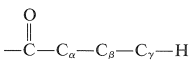In this pathway (Norrish type II process), cleavage occurs at the $$\ce{C}_\alpha \ce{-C}_\beta$$ bond to give, as the major product, a ketone of shorter chain length and an alkene. Thus for 2-pentanone:This reaction occurs in an interesting way. Whatever the nature of then $$n \rightarrow \pi^*$$ excited state, $$S_1$$ or $$T_1$$, the primary photochemical reaction is the abstraction of a hydrogen atom from the $$\gamma$$ carbon by the carbonyl oxygen to give the diradical, $$1$$:The subsequent dark reactions readily are understood as typical of diradicals. Cleavage of $$1$$ at $$\ce{C}_\alpha \ce{-C}_\beta$$ gives ethene and an enol, which rearranges to the ketone. Alternatively, $$1$$ can cyclize to a cyclobutanol:A variety of photodissociation reactions have been found to take place with ketones, but the products almost always can be explained as the result of Norrish type I and/or II cleavage. Examples are:Photoreduction of Diaryl Ketones

Diaryl ketones do not undergo photodissociation in the same way as alkyl ketones, probably because cleavage to phenyl and other aryl radicals is unfavorable (Table 4-6). Nevertheless, aromatic ketones are photochemically reactive in the presence of compounds that can donate a hydrogen atom, with the result that the carbonyl group is reduced. Indeed, one of the classic photochemical reactions of organic chemistry is the formation of 1,1,2,2-tetraphenyl-1,2-ethanediol ($$3$$, benzopinacol) by the action of light on a solution of diphenylmethanone ($$2$$, benzophenone) in isopropyl alcohol. The yield is quantitative.The light is absorbed by $$2$$ and the resulting activated ketone, $$2^*$$, removes a hydrogen from isopropyl alcohol:Benzopinacol results from dimerization of the radicals, $$4$$:Since the quantum yields of 2-propanone and benzopinacol both are nearly unity when the light intensity is not high, it is clear that two of the radicals, $$4$$, must be formed for each molecule of $$2$$ that becomes activated by light. This is possible if the 2-hydroxy-2-propyl radical formed by Equation 28-7 reacts with $$2$$ to give a second diphenylhydroxymethyl radical:This reaction is energetically favorable because of the greater possibility for delocalization of the odd electron in $$4$$ than in the 2-hydroxy-2-propyl radical.

Photochemical formation of $$3$$ also can be achieved from diphenylmethanone, $$2$$, and diphenylmethanol, $$5$$:The mechanism is similar to that for isopropyl alcohol as the reducing agent: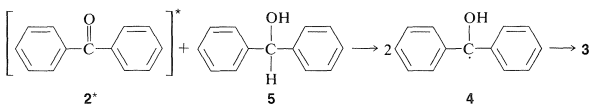This reduction is believed to involve the triplet state of $$2$$ by the following argument: Formation of $$3$$ is reasonably efficient even when the concentration of the alcohol, $$5$$ is low; therefore, whatever the excited state of the ketone, $$2^*$$, that accepts a hydrogen atom from $$5$$, it must be a fairly long-lived one. Because solutions of $$2$$ show no visible fluorescence, they must be converted rapidly to another state of longer life than the singlet $$\left( S_1 \right)$$. The long-lived state is then most reasonably a triplet state. In fact, if naphthalene is added to the reaction mixture, formation of benzopinacol, $$3$$ is drastically inhibited because the benzophenone triplet transfers energy to naphthalene more rapidly than it reacts with the alcohol, $$5$$ (see Section 28-1A).

Photochemical Isomerization of Cis and Trans Alkenes

An important problem in many syntheses is to produce the desired isomer of a cis-trans pair of alkenes. The problem would not arise if it were possible to isomerize the undesired isomer to the desired isomer. In many cases such isomerizations can be carried out photochemically. A typical example is afforded by cis- and trans-1,2-diphenylethene (stilbene):Here the trans form is easily available by a variety of reactions and is more stable than the cis isomer because it is less sterically hindered. However, it is possible to produce a mixture containing mostly cis isomer by irradiating a solution of the trans isomer in the presence of a suitable photosensitizer. This process in no way contravenes the laws of thermodynamics because the input of radiant energy permits the equilibrium point to be shifted from what it would be normally.

Isomerization appears to occur by the following sequence: The sensitizer, usually a ketone such as benzophenone or 1-(2-naphthyl)ethanone, is raised by an $$n \rightarrow \pi^*$$ transition from the singlet ground state $$\left( S_0 \right)$$ to an excited state $$\left( S_1 \right)$$ by absorption of light. Intersystem crossing then occurs rapidly to give the triplet state $$\left( T_1 \right)$$ of the sensitizer: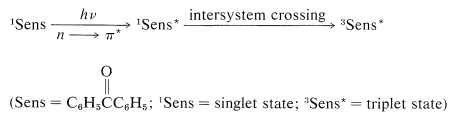The next step is excitation of the alkene by energy transfer from the triplet state of the sensitizer. Remember, the net electron spin is conserved during energy transfer, which means that the alkene will be excited to the triplet state:

$^3\text{Sens}^* \left( \uparrow \uparrow \right) + ^1\text{Alkene} \left( \uparrow \downarrow \right) \rightarrow ^1\text{Sens} \left( \uparrow \downarrow \right) + ^3\text{Alkene}^* \left( \uparrow \uparrow \right)$

The triplet state of the alkene is most stable when the $$p$$ orbitals, which make up the normal $$\pi$$ system of the double bond, are not parallel to one another (Figure 6-17). Therefore, if the energy-transfer process leads initially to a planar triplet, this is converted rapidly to the more stable nonplanar form. The excitation of either the cis or the trans isomer of the alkene appears to lead to a common triplet state, as shown in Figure 28-4.Figure 28-4: Schematic energy levels for cis- and trans-1,2-diphenylethene. The upward transitions are achieved by transfer of energy from triplet sensitizer. The downward transitions from the nonplanar stage marked with the rate constants $$k_t$$ and $$k_c$$ involve loss of thermal energy to the solvent, or phosphorescence. The lower energies assigned to the $$S_0$$ and $$T_1$$ states of trans-1,2-diphenylethene relative to the $$S_0$$ and $$T_1$$ states of the cis isomer reflect steric hindrance between the phenyl groups of the cis isomer.

The final step in the isomerization is decay of the alkene triplet to the ground state. This happens either by emission of light (phosphorescence) or by having the triplet energy converted to thermal energy without emission of light. Either way, the cis or trans isomer could be formed and, as can be seen from Figure 28-4, the ratio of isomers produced depends on the relative rates of decay of the alkene triplet to the ground-state isomers, $$k_c/k_t$$. This ratio turns out to favor formation of the less stable isomer. Therefore, provided both isomers can be photosensitized efficiently, sensitized irradiation of either one will lead ultimately to a mixture of both, in which the thermochemically less stable isomer predominates. The sensitizer must have a triplet energy in excess of the triplet energy of the alkene for energy transfer to occur, and the photostationary or equilibrium point is independent of the nature of the sensitizer when the latter transfers energy efficiently to both cis and trans isomers. In the practical use of the sensitized photochemical equilibrium of cis and trans isomers, it is normally necessary to carry out pilot experiments to determine what sensitizers are useful.

Another example of how photochemical isomerization can be used is provided by the equilibration of the $$E$$ and $$Z$$ form of 1-bromo-2-phenyl-1-propene:The $$E$$ isomer is formed to the extent of $$95\%$$ in the dehydrohalogenation of 1,2-dibromo-2-phenylpropane:Photoisomerization of the elimination product with 1-(2-naphthyl)ethanone as sensitizer produce a mixture containing $$85\%$$ of the $$Z$$ isomer.

Photochemical Cyclization Reactions

One may well ask why the isomerization of alkenes discussed in the preceding section requires a sensitizer. Why cannot the same result be achieved by direct irradiation? One reason is that a $$\pi \rightarrow \pi^*$$ singlet excited state $$\left( S_1 \right)$$ produced by direct irradiation of an alkene or arene crosses over to the triplet state $$\left( T_1 \right)$$ inefficiently (compared to $$n \rightarrow \pi^*$$ excitation of ketones). Also, the $$S_1$$ state leads to other reactions beside isomerization which, in the case of 1,2-diphenylethene and other conjugated hydrocarbons, produce cyclic products. For example, cis-1,2-diphenylethene irradiated in the presence of oxygen gives phenanthrene by the sequence of Equation 28-8. The primary photoreaction is cyclization to a dihydrophenanthrene intermediate, $$6$$, which, in the presence of oxygen, is converted to phenanthrene:The cyclization step of Equation 28-8 is a photochemical counterpart of the electrocyclic reactions discussed in Section 21-10D. Many similar photochemical reactions of conjugated dienes and trienes are known, and they are of great interest because, like their thermal relatives, they often are stereospecific but tend to exhibit stereochemistry opposite to what is observed for formally similar thermal reactions. For example,These reactions are $$4n$$-electron concerted processes controlled by the symmetry of the reacting orbitals. The thermal reaction is most favorable with a Mobius transition state (achieved by conrotation), whereas the photochemical reaction is most favorable with a Huckel transition state (achieved by disrotation).

Conjugated dienes also undergo photochemical cycloaddition reactions. Related thermal cycloadditions of alkadienes have been discussed in Sections 13-3A, 21-10A, and 21-10D, but the thermal and photochemical reactions frequently give different cyclic products. Butadiene provides an excellent example of the differences:In the thermal reaction the [4 + 2] or Diels-Alder adduct is the major product, whereas in the photochemical reaction [2 + 2] cycloadditions dominate. Because the photochemical additions are sensitized by a ketone, $$\ce{C_6H_5COCH_3}$$, these cycloadditions occur through the triplet state of 1,3-butadiene and, as a result, it is not surprising that these cycloadditions are stepwise, nonstereospecific, and involve diradical intermediates.

Excitation:Direct irradiation of 1,3-butadiene with $$254$$-$$\text{nm}$$ light produces cyclobutene and small amounts of bicyclo[1.1.0]butane along with dimers.

In contrast to conjugated dienes, simple alkenes such as 2-butene do not react easily by photosensitized cycloaddition. But they will form [2 + 2] cycloadducts on direct irradiation. These additions occur by way of a singlet excited state and are stereospecific:A related reaction, which has no precedent in thermal chemistry, is the cycloaddition of an alkene and an aldehyde or ketone to form an oxacyclobutane:In this kind of addition the ground-state alkene $$\left( S_0 \right)$$ reacts with an excited state (usually $$T_1$$) of the carbonyl compound by way of a diradical intermediate: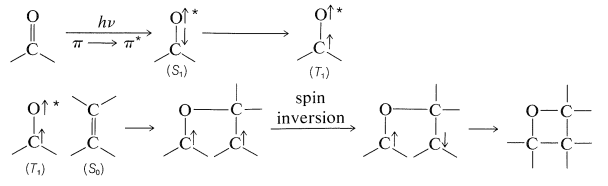Singlet Oxygen Reactions

The ground state of molecular oxygen is unusual because it is a triplet state. Two electrons of parallel spin occupy separate $$\pi$$ orbitals of equal energy (degenerate), as shown schematically in Figure 28-5,$$^3$$ The next two higher electronic states both are singlet states and lie respectively $$24$$ and $$37 \: \text{kcal mol}^{-1}$$ above the ground state. From this we can understand why ordinary oxygen has the properties of a diradical and reacts rapidly with many radicals, as in the radical-chain oxidation of hydrocarbons (autoxidation; Sections 15-10 and 16-9E):Figure 28-5: Electronic configurations of the two highest occupied (degenerate) $$\pi$$ orbitals of oxygen $$\left( O_2 \right)$$ in the ground and excited states.

Oxygen also efficiently quenches excited triplet states of other molecules $$\left( ^3A^* \right)$$ and, in accepting triplet energy, is itself promoted to an excited singlet state. Notice that the total spin orientation is conserved:Singlet oxygen is highly reactive toward many organic molecules and will form oxygenated addition or substitution products. As one example, conjugated dienes react with singlet oxygen to give peroxides by [4 + 2] cycloaddition. Because only singlet states are involved, this addition is quite analogous to thermal Diels-Alder reactions (Section 21-10A):If the alkene or alkadiene has at least one hydrogen on the carbon adjacent to the double bond, reaction with singlet oxygen may give hydroperoxides. The mechanism of this reaction is related to [4 + 2] cycloadditions and is presumed to occur through a Huckel pericyclic transition state (see Section 21-10D):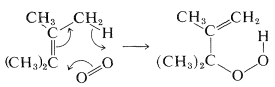Many reactions of this type can be achieved by allowing the hydrocarbon to react with oxygen in the presence of a sensitizing dye that strongly absorbs visible light. The dyes most commonly used for this purpose include fluorescein (and its chlorinated analogs, eosin and rose bengal), methylene blue, and porphyrin pigments (such as chlorophyll).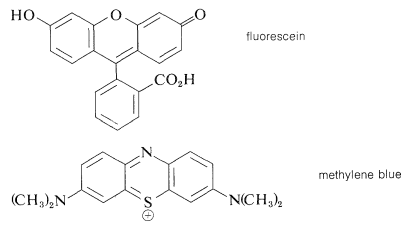The overall process of photosensitized oxygenation of a substrate $$\left( \ce{A} \right)$$ proceeds by the following steps:

$^1\text{Sens} \overset{h \nu}{\longrightarrow} \: ^1\text{Sens}^* \longrightarrow ^3\text{Sens}^*$

$^3\text{Sens}^* + ^3\ce{O_2} \longrightarrow ^1\text{Sens} + ^1\ce{O_2}*$

$^1\ce{O_2}* + \ce{A} \longrightarrow \ce{AO_2}$

Singlet oxygen can be produced chemically as well as by photochemical sensitization. There are several chemical methods available, one of the best known being the reaction of sodium hypochlorite with peroxide:

$\ce{NaOCl} + \ce{H_2O_2} \rightarrow \ce{NaCl} + \ce{H_2O} + ^1\ce{O_2} \tag{28-9}$

An alternative method of formation, which can be used in organic solvents at low temperatures, involves the thermal decomposition of triethyl phosphite ozonide (Equation 28-10):Regardless of whether singlet oxygen is formed chemically or photochemically, it gives similar products in reactions with alkenes.

Photobiology

Photosensitized reactions of oxygen are largely damaging to living organisms. Indeed, singlet oxygen reacts destructively with amino acids, proteins, and nucleic acids. How does an organism protect itself against the damaging effects of oxygen? There are no simple answers, but green plants provide a clue. Chlorophyll is an excellent sensitizing dye for singlet oxygen; yet green plants evidently are not harmed because of it. A reason may be that singlet oxygen is quenched very efficiently by other plant pigments, especially the carotenoid pigments such as $$\beta$$-carotene (Section 2-1). That this is the case is indicated by the fact that mutant plants unable to synthesize carotene are killed rapidly by oxygen and light.

That direct irradiation with ultraviolet light is damaging to single-cell organisms is well known. It also is known that the nucleic acids, DNA and RNA, are the important targets of photochemical damage, and this knowledge has stimulated much research in the field of photobiology in the hope of unravelling the chemistry involved.

An interesting and significant outcome is the finding that the pyrimidine bases of nucleic acids (uracil, thymine, and cytosine) are photoreactive and undergo [2 + 2] cycloadditions on irradiation with ultraviolet light. Thymine, for example, gives a dimer of structure $$7$$:Comparable experiments with the nucleic acids have confirmed that cycloaddition of their pyrimidine bases also occurs with ultraviolet light and effectively cross-links the chains, a process obviously quite inimical to the functioning of the DNA (Section 25-13B). A remarkable and not well understood aspect of photobiology is the repair and defense mechanism both plants and animals possess to minimize the damaging effects of radiation.

On the positive side, there are photochemical reactions that are essential for human health. One of these is the formation of vitamin D (the antirachitic vitamin) by irradiation of ergosterol. This photochemical reaction is an electrocyclic ring opening of the cyclohexadiene ring of ergosterol of the type described in Section 28-2D. The product, previtamin D2, subsequently rearranges thermally to vitamin D2: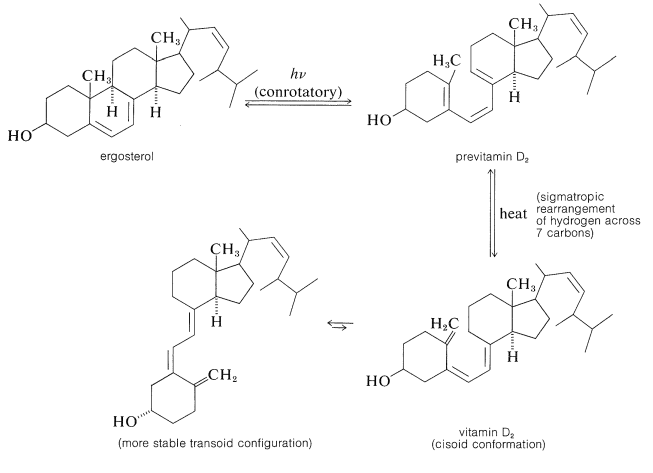$$^1$$The einstein unit is defined in Section 9-4.

$$^2$$Recipient with G. Porter of the Nobel Prize in chemistry in 1967 for work on photochemical reactions.

$$^3$$For a more detailed account of the electronic configuration of molecular oxygen, see M. Orchin and H. H. Jaffe, The importance of Antibonding Orbitals, Houghton Mifflin Co., Boston, 1967; or H. B. Gray, Chemical Bonds, W. A. Benjamin, Inc., Menlo Park, Calif., 1973.